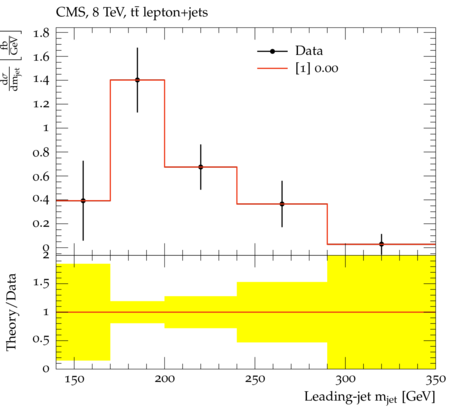### CMS_2017_I1518399

Back to index

Measurement of the differential and normalised differential $t\bar{t}$ production cross section as a function of the leading jet mass. The measurement was performed in the lepton+jets channel and the fiducial measurement phase space is enriched with events where the leading jet includes all decay products of a hadronically decaying top quark. This analysis is to be run on ${t\bar{t}}$ simulation. The objects are defined as follows - Lepton: electron or muon originating from the decay of a W boson - Jets: jets are clustered from final state particles using the Cambridge-Aachen algorithm with a distance parameter of 1.2. If a lepton overlaps with a jet (distance in the eta-phi plane smaller 1.2) its four momentum is subtracted from the four momentum of the jet. Definition of the particle level phase space: - only ${\rm t\bar{t}}$ decays with one top quark decaying hadronically and one top quark decaying leptonically including an electron or muon from the W decay. - lepton $\pt > 45\;\text{GeV}$ and $|\eta| < 2.1$ - at least one jet with $\pt > 400\;\text{GeV}$ and $|\eta| < 2.5$ - a second jet with $\pt > 150\;\text{GeV}$ and $|\eta| < 2.5$ - veto on additional jets with $\pt > 150\;\text{GeV}$ and $|\eta| < 2.5$ - distance between the second jet and the lepton in the eta-phi plane $\Delta R(jet2, lepton)$ smaller 1.2 - invariant mass of the leading jet larger than the invariant mass of the combination of the second jet and lepton The differential and normalised differential cross section was measured as a function of the leading jet mass.

CMS_2017_I1518399_d01-x01-y01:Generated at Sunday, 28. January 2018 12:08PM